Governing equations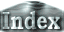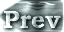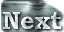The non-dimensional governing equations are those of a two-dimensional Boussinesq fluid with the topographic b effect caused by the inclined boundaries (Busse,1986).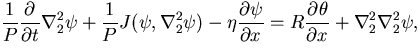(1)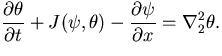(2)

y and q are stream function and temperature disturbance. Non-dimensional numbers appearing in the system are the Rayleigh number R, the Prandtl number P, and the parameter of topographic b effect h. Further explanation of these equations is given in Appendix A.

The fixed heat flux condition is given as the thermal boundary condition.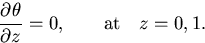(3)

The kinematic and dynamic boundary conditions are impermeable and free-slip.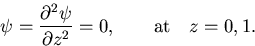(4)

 Governing equations